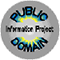PD InfoPublic Domain Information Project

Public Domain Sheet Music Books# Ragtime Rarities

Out of Print

• Used Copies Usually in Stock - Call for Price 770-993-8074
• Also check Amazon.com - eBay - Used Book Sellers
• Selected by and Introduction by Trebor Jay Tichenor
• xiv + 396 pages" x 12"Paperback
• Dover Publications, Inc.
• ISBN: 0486231577

Sheet Music reprints of first or early editions of 63 piano rags from 1897 to 1917.   Most of these works are relatively unknown, but are a joy for listening and playing.  All are piano rags and do not have lyrics.

PUBLIC DOMAIN STATUS: All selections are reprints of original or early editions with copyright date and publisher shown.  All are believed to be in the public domain in the United States.   Public domain status may not apply in countries outside of the United States where copyright protection may exist.

SONGS INCLUDED:  =][=  African Pas' (1902)  =][=  Alabama Dream (1899)  =][=  Bantam Step (1916)  =][=  Big Foot Lou (1899)  =][=  The Black Cat Rag (1905)  =][=  Bunch o' Blackberries (1899)  =][=  Cactus Rag (1916)  =][=  Car-Barlick-Acid (1903)  =][=  Chimes (1911)  =][=  Cleanin' Up in Georgia (1899)  =][=  The Climbers Rag (1911)  =][=  Cole Smoak (1906)  =][=  Coon Hollow Capers (1899)  =][=  A Cotton Patch (1902)  =][=  Dixie Blossoms (1906)  =][=  Doc Brown's Cake Walk (1899)  =][=  Dusty Rag (1908)  =][=  Echoes from the Snowball Club (1898)  =][=  Eli Green's Cake Walk (1898)  =][=  Encore Rag (1912)  =][=  The Fascinator (1903)  =][=  Felix Rag (1910)  =][=  Flying Arrow (1906)  =][=  Glad Cat Rag (1905)  =][=  Impecunious Davis (1899)  =][=  Jinx Rag (1915)  =][=  Jungle Time (1909)  =][=  Just Ask Me (1902)  =][=  The Lion Tamer Rag (1913)  =][=  Louisiana Rag (1897)  =][=  Ma Rag Time Baby (1898)  =][=  Majestic Rag (1914)  =][=  Mandy's Broadway Stroll (1898)  =][=  Mashed Potatoes (1911)  =][=  Medic Rag (1910)  =][=  On the Pike (1904)  =][=  One o' Them Things (1904)  =][=  The Original Chicago Blues (1915)  =][=  Peaceful Henry (1901)  =][=  Peaches and Cream (1905)  =][=  The Queen Rag (1911)  =][=  Rag Pickers Rag (1904)  =][=  Ragtime Rags (1898)  =][=  Shake Yo' Dusters! or Piccaninny Rag (1898)  =][=  The Sheath (1908)  =][=  The Smoky Topaz (1901)  =][=  Southern Rag Medley 1 (1908)  =][=  Southern Rag Medley 2 (1909)  =][=  Sponge (1911)  =][=  St. Louis Tickle (1904)  =][=  The Stinging Bee (1908)  =][=  Summer Breeze, A (1903)  =][=  Sure Fire Rag (1910)  =][=  Sweet Pickles (1907)  =][=  A Tennessee Jubilee (1899)  =][=  That Demon Rag (1912)  =][=  That Hand Played Rag (1914)  =][=  The Thriller Rag (1909)  =][=  Tom and Jerry Rag (1917)  =][=  The Watermelon Trust (1906)  =][=  Whittling Remus (1900)  =][=  Whoa! Nellie! (1915)  =][=  X. L. Rag (1903)  =][=

### What We Do . . .770-993-8074